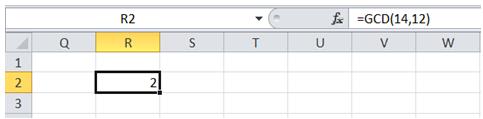## Find greatest common divisor in Excel

To do it in Excel, here is the answer:

a) Enter the formula =GCD(14,12) as shown below where 14 and 12 are the dimensions of the room.

GCD stands for "Greatest Common Divisor" and returns the largest positive integer that divides both 14 and 12 (that is fed as arguments in this case).